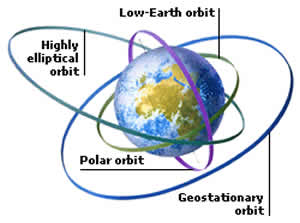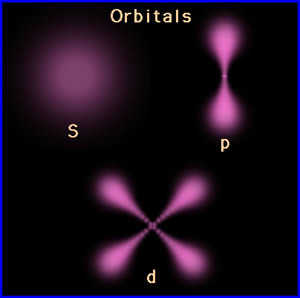# Difference between Orbit and Orbital

##### Key Difference: An orbit is a curved path that is taken by an object around a star, planet, moon, etc. An orbital is a shape resulting in the combination of all probable orbits. It is an indecisive region inside an atom within which the possibility to find an electron(s) is highest.People often get confused with the words orbit and orbital. They sound as if they are similar to each other, but they aren't! Orbit is the path traced by an electron revolving around the nucleus of an atom, whereas, any particular electron will be found in a region of space is known as an orbital.

The paths on which the planets revolve are called as orbits. In the astronomical studies, orbit is the more discussed term. It is a two-dimensional rounded path in a space, which represents the planet movement of electrons around the nucleus. An orbit is a curved path that is taken by an object around a star, planet, moon, etc. Thus, one can simply define it "as the path determining the motion of a body around the other body". The most common example is our solar system in which planets like Earth, Saturn and Jupiter follow an orbit around the sun.

There are two types of Orbits: Geosynchronous Orbit and Geostationary Orbit. A geosynchronous orbit is an orbit around a planet which has the same orbital period as the planet’s rotation period. Whereas, geostationary orbit is a kind of geosynchronous orbit but with an addition feature. It remains stationary with respect to a single point on the surface of the planet.On the Dictionary.com, the definition of orbital is "a wave function describing the state of a single electron in an atom (atomic orbital) or in a molecule (molecular orbital)." and also "the wave function of an electron in an atom or molecule, indicating the electron's probable location." It is a region of space around the nucleus where the probability of finding an electron is maximum. An orbital is an indecisive region inside an atom within which the possibility to find an electron(s) is highest. Orbitals arise in different forms and abilities in accordance with the component and its atomic number.

Comparison between Orbit and Orbital:

 Orbit Orbital Description The paths on which the planets revolve are called orbits. An orbital is a shape resulting in the combination of all probable orbits. In simple terms It is well-defined circular path followed by electron around nucleus. It is a region of space around the nucleus where the probability of finding an electron is maximum. Word Noun Adjective It signifies Two dimensional motion nation of electron around nucleus. Three dimensional motion of electron around nucleus. Accommodate An orbit can accommodate 2n² electrons in it. Orbital can accommodate 2, 6, 10 and 16 electrons. It is categorized with s, p, d and f type orbital. Types Low earth orbits Highly elliptical orbits Geosynchronous orbit Geostationary orbit Polar orbit Molecular orbital Atomic orbital Hybrid orbital Complex orbital Real orbital Exists Exists for any two bodies having some mass. Exists only for an atom and an electron. It is a notion It is a notion from celestial mechanisms and astronomy. It is a notion from quantum mechanisms, spectrometry, and chemistry. Shape Rounded in shape. Contain different shapes. Directional characteristics No directional characteristics Except s-orbitals (The electron distribution is spherically symmetrical in an s-orbital around the nucleus) others have directional characteristics.

Image Courtesy: factmonster.com, iun.edu